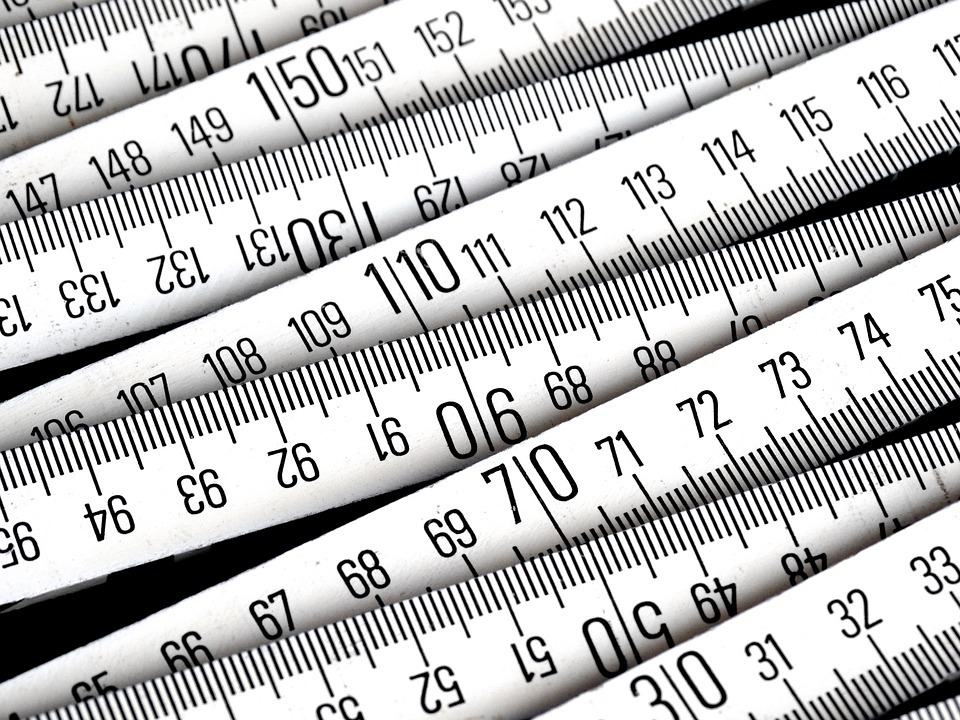# Square Feet and Square Metres. What’s the Difference and Which is More Widely Used?Usually when we talk about measurements of properties, be it residential, commercial or industrial properties, we may come across terms such as square feet and square metres which are commonly used units to measure sizes of properties.

## Square Feet

This term is possibly one of the most commonly used terms which you may have also heard or seen many times before when it comes to real estate in Singapore. Square foot can also be known as an area equal to a square which measures one foot long on each side. It is sometimes also written as sq ft in short.

## Square Metres

We may sometimes also hear people using square metres as a form of measurement as certain profile of buyers or tenants are used to square metres back in their home country. Square metre can also be known as an area equal to a square which measures one metre long on each side. It is sometimes also known as sq m in short.

## Difference Between Square Feet & Square Metres

Both square meter and square feet measure the area of a square. According to research, the difference is that square metres seem to be used more frequent internationally with the exception of some countries such as the US and Canada which often use square feet instead of square metres.

## Formula for Conversion

As we know, 1 metre is approximately 3.28 feet. When converting from square metres to square feet, you may multiply the size in square metres by 10.76 to arrive at the approximate size in square feet. The reason why we included the word approximate is because there are still more decimal places which we did not take into account for easy calculation and illustration purposes.

1 square metre = 3.28 feet x 3.28 feet = 10.76 square feet (approximately)

Therefore 1 square metre approximately equals to 10.76 square feet

For example, if the size is 150 square metres, the approximate calculations will be as follows :

150 square metres x 10.76 = 1,614 square feet (approximately)

## Why It is Good to Know

Sometimes when we go around Singapore or when looking out for overseas or international properties, we may come across some people using square feet while some use square metres. In order to imagine or have an idea what the size is like, you may want to convert sizes from square metres to square feet or from square feet to square metres. By doing so, you will be able to have a rough idea what the size of the property is like as compared to our current home be it hdb, condo or landed properties.

## Which is More Widely Used in Singapore

Based on our understanding and interactions with locals in Singapore, square feet seems to be the more widely used unit of measurement. For example, whenever we talk about hdb, condominiums or landed, warehouses or factories, we tend to use square feet more often. This may be why most property portals often use square feet as the main unit of measurement. However, there will be some who prefer using square metres.

By having the formula for conversion in mind, it would not be difficult to do a quick conversion from square metres to square feet and vice versa. By knowing this, it would also not matter that much whichever unit of measurement is more widely used since we can multiply or divide using the right formula.